﻿ Windows > Calculator > Parts of the Calculator

# Parts of the CalculatorThe Calculator has several parts: a keypad, an input area, a preview area, and pop-up menus for inserting special mathematical elements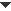Keypad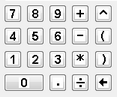Click the buttons in the Calculator’s keypad to insert numbers, decimal points, operators, and parentheses into your calculation or function. Instead of clicking one of these buttons, you can type the corresponding key on your computer’s keyboard. (Use the / key — the forward slash — on the keyboard for division.) Click the left-arrow key on the keypad, or the Backspace key (Windows) or Delete key (Macintosh) on the keyboard, to delete the last item you typed. If you’re using the Calculator to define a function, you can click the key labeled x to enter the value of the independent variable. The x key is present only when you’re defining a function; it changes to y, θ, or r depending on the form of the equation.Input Area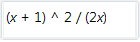The input area displays each element you insert into the calculation or function as you enter it. Refer to the input area to see exactly what you’ve entered. You can also click in the input area or press the right- or left-arrow key on the keyboard to change the insertion point and add new numbers, operators, and so forth in the middle of an existing expression. If the input area does not form a valid mathematical expression, the portion of the input area up to the first error is shown in black. The portion following the first error appears in red.Preview Area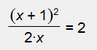The preview area shows a mathematically formatted preview of the input area when it contains a valid expression. Use the preview to be sure that you’ve entered the desired calculation or function correctly — that you have the parentheses in the right places and that you have the correct order of operations.Pop-up Menus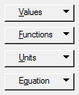Depending on whether you’re defining a calculation or a function, either three or four pop-up menus appear. The last of these, the Equation pop-up menu, appears only when you’re defining or editing a function rather than a calculation.

You can click an existing value or function in the sketch to insert it into the Calculator. You can also click a Hot Text link to a value or function to enter it.Values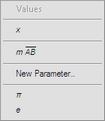This pop-up menu allows you to enter the value of any selected measurement in the sketch, to insert a new parameter, or to insert the value of the constant π or e. The values that appear in this menu include measurements or calculations that were selected in the sketch when the Calculator was opened. If you want to use a value from the sketch that doesn’t appear in this menu, click the value in the sketch to insert it into your expression. (If the value is hidden behind the Calculator, drag the Calculator by its title bar to move it out of the way and then click the value.) When you edit a calculation or function, you can insert only values that don’t depend on the object you’re editing. In other words, if you’re editing a calculated value 2·AB, and your sketch has another calculation which uses this result to calculate 2·AB + 2·CD, you cannot insert the value of the second calculation into the first. If you’re defining a function, you can also use the Values pop-up menu to insert the value of the independent variable x, y, r, or θ.Functions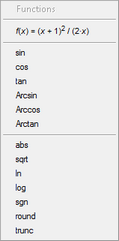This pop-up menu allows you to use in your expression any selected function you’ve already defined in the sketch, or to use any of Sketchpad’s standard functions. Sketchpad’s standard functions include the trigonometric functions, the inverse trig functions, and these additional functions:

 abs absolute value sqrt square root ln natural logarithm (base e) log common logarithm (base 10) sgn signum (returns +1, 0, or -1 depending on whether its argument is positive, zero, or negative) round round (rounds its argument to the nearest whole number) trunc truncate (truncates its argument by removing the fractional part For example, trunc(2.5) = 2, and trunc(–7.8) = –7.)

The sgn function is especially useful in creating a calculation that makes a decision based on the value of a variable, measurement, or parameter.

You can also click on an existing function that you’ve defined in the sketch to insert it into the Calculator.Units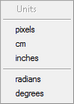The Units pop-up menu allows you to insert any desired angle or distance unit (pixels, cm, inches, radians, or degrees). A unit must be attached to a number, so the Units menu is enabled whenever you’ve just inserted a numeric constant — either an ordinary number or one of the constants π and e. See also: Plot Function New ParameterEquation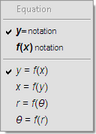The Equation pop-up menu appears only when you create a function or edit a function, and allows you to set the form in which the function plot appears. Use the first two choices to determine whether your equation appears in y= notation (for instance, y = x2) or in f(x) notation (for instance, f(x) = x2). To change the default setting for new functions, choose Use the bottom four choices to determine whether the independent variable is x, y, θ, or r. Choose y = f(x) or x = f(y) for a square or rectangular plot, or choose r = f(θ) or θ = f(r) for a polar plot.